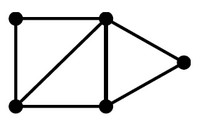# Graph - Path

A path is a sequence of edges.

How to get from a node A to a node B.

If two nodes are connected only by one path, there is no cycle

A sequence of edges $[\{x_1, x_2\}, \{x_2, x_3\}, \{x_3, x_4\}, \dots , \{x_{k_1}, x_k\}]$ is called a $x_1-to-x_k$ path.

## Example

• one goes through “Wriston Quad” ,
• one goes through “Keeney Quad”

## Documentation / Reference

Discover MoreGraph (Network - Nodes and edges)

A graph is a set of vertices connected by edges. See Data representation that naturally captures complex relationships is a graph (or network). Except of the special graph that a tree is, the data...Graph - (Edge | Links | Arcs | Lines | Arrows) - Association

An edge model a relationship betweentwo node in a graph. Every edge model therefore a binary relationship (relationship between two elements) . An Edge is also known as: Links Arcs Lines Arrows...Graph - Acyclic

Acyclic means that any two vertices (node) are connected by exactlyone path. There is no cycleGraph - Cycle

A path that starts from a node and can come back to the same node is called a cycle A graph without a cycle is called acyclic.Graph Analysis - Shortest path (Path Finding)

How to identify the path that best meets some criteria (shortest, cheapest, fastest, etc) between two points in a large network. ... celue/status/824197949588697088/photo/1Pat Eskinay ...